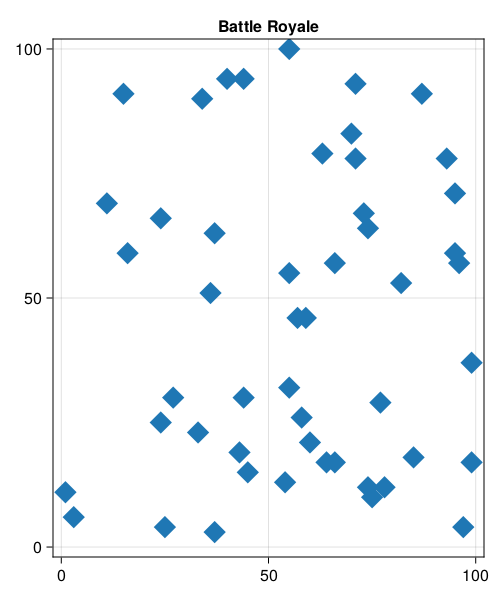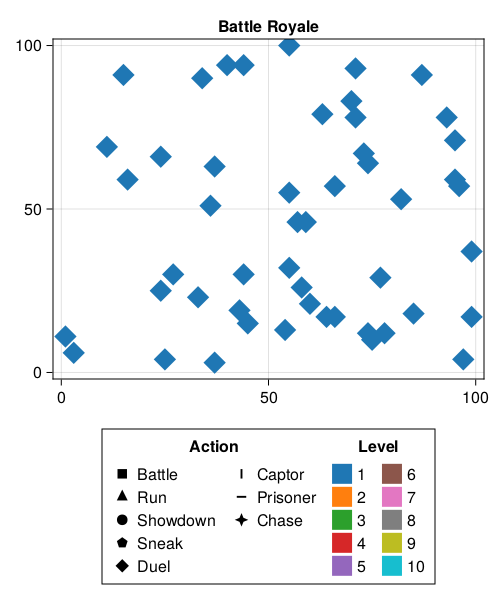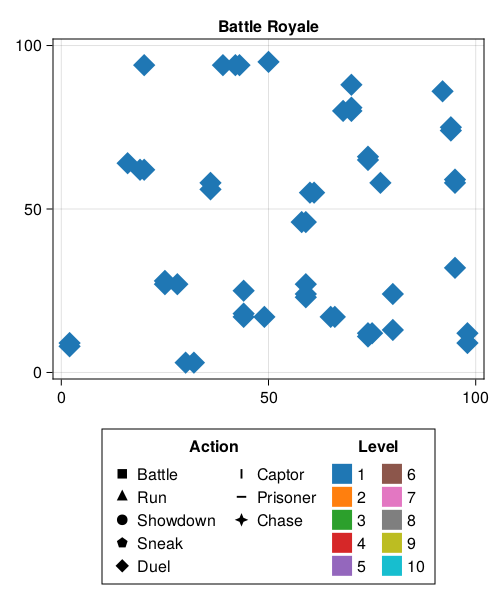# Battle Royale

This example illustrates how to leverage higher dimensions of a GridSpace to identify the distance from neighbors not just spatially, but also categorically. We'll also use the walk! function extensively.

## Rules of Engagement

Agents wander around the map looking for opponents. When a grid space is occupied by two or more agents there will be blood. With experience gained from the fight, the victor searches for more opponents to crush and losers scurry away defeated or possibly even die. This process repeats until there is a single, definitive winner.

For this battle ground to exist, the following rules must be followed:

• Agents have an experience level, starting at level 1 up to a maximum of 10.
• Agents will search for the nearest worthy opponent (one with equal or ±1 experience level) and move towards them to attack, so long as something more important doesn't happen, which could be
• A tougher opponent (with experience level +2 or higher) is nearby: run!
• There are no worthy opponents available, but there are weak ones (with experience level -2 or lower): chase them down.
• Capture and taunt a weaker opponent, then kill them.
• Notice a tough opponent is occupied, sneak up and kill them.
• There is no-one worthy to fight, but also no-one left to taunt. All bets are off: THERE CAN BE ONLY ONE.

Battles are won by weighted chance - a higher level gives an agent a larger chance of winning, but does not guarantee it. When a victor is chosen

• The difference in experience between opponents is swapped.
• If an agents experience reaches 0, they die.

Captured opponents will be killed once taunted. The captor will gain half of their experience. If an opportunist manages to take the captor by surprise, they can gain up to half of the captor's experience. This means a level 1 agent may eliminate a level 10 captor and jump straight to level 6.

Once all rules of engagement have been exhausted, the final showdown begins. Opponents fight their closest adversary regardless of experience level. Winner takes all.

## Model Setup

using Agents
using Random
using InteractiveDynamics
using CairoMakie

@agent Fighter GridAgent{3} begin
has_prisoner::Bool
capture_time::Int
shape::Symbol # shape of the fighter conveys what action they are currently doing
end

As you can see, the properties of out agent are very simple and contain only two parameters that are needed to store context from one time step to the next. All other properties needed are stored in the space. pos is three-dimensional, two for the actual space agents move within, and a third categorical dimension representing their level.

Now let's set up the battle field:

function battle(; fighters = 50, seed = 6547)
model = ABM(
Fighter,
GridSpace((100, 100, 10); periodic = false);
scheduler = Schedulers.randomly,
rng = Random.Xoshiro(seed),
)

n = 0
while n != fighters
pos = (rand(model.rng, 1:100, 2)..., 1) # Start at level 1
if isempty(pos, model)
n += 1
end
end

return model
end

model = battle()
StandardABM with 50 agents of type Fighter
space: GridSpace with size (100, 100, 10), metric=chebyshev, periodic=false
scheduler: randomly

50 opponents positioned randomly on a 100x100 grid, with no escape (periodic = false). To leverage categorical dimensions fully, non-periodic chebyshev space is necessary.

## Game Dynamics

To implement the rules of engagement, only an agent_step! function is required, along with a few helper functions.

loc(agent) = agent.pos[1:2]
level(agent) = agent.pos
level (generic function with 1 method)

space allows us to invoke a number of helpful utilities provided by Agents.jl but only operate on our spatial dimensions, level is a wrapper to access the agent's experience easily.

Nearest agents that satisfy our search criteria can be identified via Euclidean distance solely on the spatial dimensions of our GridSpace.

function closest_target(agent::Fighter, ids::Vector{Int}, model::ABM)
if length(ids) == 1
closest = ids
else
close_id = argmin(map(id -> edistance(loc(agent), loc(model[id]), model), ids))
closest = ids[close_id]
end
return model[closest]
end
closest_target (generic function with 1 method)

Since our battles are only between opponents with equal, or as much as one level apart, the odds can be set explicitly. Stronger opponents have twice the capacity of winning a match.

function battle!(one::Fighter, two::Fighter, model)
if level(one) == level(two)
# Odds are equivalent
one_winner = rand(model.rng) < 0.5
elseif level(one) > level(two)
# Odds are in favor of one
one_winner = 2 * rand(model.rng) > rand(model.rng)
else
# Odds are in favor of two
one_winner = rand(model.rng) > 2 * rand(model.rng)
end

one_winner ? (up = one; down = two) : (up = two; down = one)

new_lvl_up = min(level(up) + 1, 10)
new_pos_up =
clamp.(rand(model.rng, -1:1, 2) .+ loc(up), [1, 1], size(model.space)[1:2])
move_agent!(up, (new_pos_up..., new_lvl_up), model)
new_lvl_down = level(down) - 1
if new_lvl_down == 0
kill_agent!(down, model)
else
move_agent!(down, (loc(down)..., new_lvl_down), model)
end
end
battle! (generic function with 1 method)

If an agent has a prisoner, it will taunt it for a time, then kill it, so long as an opportunist doesn't sneak up on them first! Here we use the tuple constructor with nearby_ids to look for agents at the same position as the captor (0, 0), and any level (..., 10). We could also use the range constructor in this instance nearby_ids(agent, model, [(1, 0:0), (2, 0:0)]), meaning which is more performant but not as readable.

function captor_behavior!(agent, model)
close_ids = collect(nearby_ids(agent, model, (0, 0, 10)))
if length(close_ids) == 1
# Taunt prisoner or kill it
prisoner = model[close_ids]
if prisoner.capture_time > 10
agent.shape = :rect
gain = ceil(Int, level(prisoner) / 2)
new_lvl = min(level(agent) + gain, 10)
kill_agent!(prisoner, model)
agent.has_prisoner = false
move_agent!(agent, (loc(agent)..., new_lvl), model)
end
else
# Someone is here to kill the captor. Could be more than one opponent
prisoner = [model[id] for id in close_ids if model[id].capture_time > 0]
exploiter = rand(
model.rng,
[
model[id]
for
id in close_ids if
model[id].capture_time == 0 && model[id].has_prisoner == false
],
)
exploiter.shape = :rect
gain = ceil(Int, level(agent) / 2)
new_lvl = min(level(agent) + rand(model.rng, 1:gain), 10)
kill_agent!(agent, model)
move_agent!(exploiter, (loc(exploiter)..., new_lvl), model)
# Prisoner runs away in the commotion
prisoner.shape = :utriangle
prisoner.capture_time = 0
walk!(prisoner, (rand(model.rng, -1:1, 2)..., 0), model)
end
end
captor_behavior! (generic function with 1 method)

When there are only few fighters standing, the stakes are higher. Prior experience is paramount since there is no gain, and fights are to the death.

function endgame!(agent, model)
origin = loc(agent)
end_ids = collect(Iterators.filter(
id -> model[id].shape == :circle && id != agent.id,
allids(model),
))
agent.shape = :circle
if !isempty(end_ids)
opponent = closest_target(agent, end_ids, model)
target = loc(opponent)
if origin == target
# Battle
agent.shape = :rect
opponent.shape = :rect
showdown!(agent, opponent, model)
else
walk!(agent, (sign.(target .- origin)..., 0), model)
end
end
end

function showdown!(one::Fighter, two::Fighter, model)
if level(one) == level(two)
# Odds are equivalent
one_winner = rand(model.rng) < 0.5
elseif level(one) > level(two)
# Odds are in favor of one
one_winner = level(one) - level(two) * rand(model.rng) > rand(model.rng)
else
# Odds are in favor of two
one_winner = rand(model.rng) > level(two) - level(one) * rand(model.rng)
end

one_winner ? kill_agent!(two, model) : kill_agent!(one, model)
end
showdown! (generic function with 1 method)

The rest of our interactions flow down a hierarchy, so we'll place them directly in the agent_step! function. We use the tuple search for occupied_ids here, as we did with close_ids above. The rest of the searches however use the range search to provide a more precise criteria.

The easiest context to explore is worthy_ids: all we want to do is find an agent with a similar level. If we used the tuple search here, we would have to search (100, 100, 1) - even though we are not at all interested in the spatial location of the neighbors at this time. (3, -1:1) is therefore more accurate representation.

A more complex example is that of strong_ids. We are looking for agents with a level 2-4 points higher withing a distance of (5, 5). The range search becomes a little verbose, but precise. An equivalent tuple search is not completely possible however. The closest solution is (5, 5, 4), which also looks for weaker opponents and must be filtered to the correct neighbor set after the fact. In this instance the range search has significant performance gains.

function agent_step!(agent, model)
if agent.capture_time > 0
# Captured agents are powerless, but we need to keep track of how long
# they have been in this state
agent.capture_time += 1
elseif agent.has_prisoner
captor_behavior!(agent, model)
else
origin = loc(agent)
# Find agents that have captives, they are not focused
occupied_ids = collect(Iterators.filter(
id -> model[id].has_prisoner,
nearby_ids(agent, model, (7, 7, 10)),
))
if !isempty(occupied_ids)
# Sneak up behind them
target = loc(closest_target(agent, occupied_ids, model))
agent.shape = :pentagon
walk!(agent, (sign.(target .- origin)..., 0), model)
else
# Opponents that are greatly higher in rank that the current agent
strong_ids = collect(nearby_ids(agent, model, [(1, -5:5), (2, -5:5), (3, 2:4)]))
if !isempty(strong_ids)
# Run away from nearest
target = loc(closest_target(agent, strong_ids, model))
agent.shape = :utriangle
walk!(agent, (sign.(origin .- target)..., 0), model)
else
# There are no distractions. Search for the closest worthy opponent
worthy_ids = collect(nearby_ids(agent, model, [(3, -1:1)]))
if !isempty(worthy_ids)
opponent = closest_target(agent, worthy_ids, model)
target = loc(opponent)
if origin == target
# Battle
agent.shape = :rect
opponent.shape = :rect
battle!(agent, opponent, model)
else
# Move towards worthy opponent
agent.shape = :diamond
walk!(agent, (sign.(target .- origin)..., 0), model)
end
else
# Find any weak targets in the vicinity
weak_ids = collect(nearby_ids(
agent,
model,
[(1, -10:10), (2, -10:10), (3, -4:-2)],
))
if !isempty(weak_ids)
prisoner = closest_target(agent, weak_ids, model)
target = loc(prisoner)
if origin == target
# Capture and taunt target
agent.has_prisoner = true
agent.shape = :vline
prisoner.capture_time += 1
prisoner.shape = :hline
else
# Chase down nearest (can move 2 steps at a time!)
agent.shape = :star4
walk!(agent, (2 .* sign.(target .- origin)..., 0), model)
end
else
# Abandon honour. This is the end
endgame!(agent, model)
end
end
end
end
end
return
end
agent_step! (generic function with 1 method)

## Let the Battle Begin

We need to write entirely custom plotting here, because we have a 3D space (that would normally be plotted as 3D), but we actually only want to plot the first two dimensions of the space. Thankfully, the infastructure of ABMObservable makes this straightforward.

abmobs = ABMObservable(model; agent_step!)
modelobs = abmobs.model
Observable(StandardABM with 50 agents of type Fighter
space: GridSpace with size (100, 100, 10), metric=chebyshev, periodic=false
scheduler: randomly)


First, we make the positions, colors and markers observables for the agents

by_id = Schedulers.ByID()
pos = lift(m -> [Point2f(m[id].pos, m[id].pos) for id in by_id(m)], modelobs)
colors = lift(m -> [ac(m[id]) for id in by_id(m)], modelobs)
am(a) = a.shape
markers = lift(m -> [am(m[id]) for id in by_id(m)], modelobs)
Observable([:diamond, :diamond, :diamond, :diamond, :diamond, :diamond, :diamond, :diamond, :diamond, :diamond  …  :diamond, :diamond, :diamond, :diamond, :diamond, :diamond, :diamond, :diamond, :diamond, :diamond])


Next, we initialize an axis and plot them

fig = Figure(resolution = (500, 600))
ax = Axis(fig[1,1]; title = "Battle Royale")
scatter!(ax, pos; color = colors, marker = markers, markersize = 25)
e = size(model.space)[1:2] .+ 2
o = zero.(e) .- 2
xlims!(ax, o, e)
ylims!(ax, o, e)
figSeems great so far! Let's add the legend

actions = [:rect, :utriangle, :circle, :pentagon, :diamond, :vline, :hline, :star4]
label_action = ["Battle", "Run", "Showdown", "Sneak", "Duel", "Captor", "Prisoner", "Chase"]
group_action = [
MarkerElement(
marker = marker,
color = :black,
strokecolor = :transparent,
markersize = 15,
) for marker in actions
]
group_level = [
PolyElement(color = color, strokecolor = :transparent) for color in cgrad(:tab10)[1:10]
]

Legend(
fig[2, 1],
[group_action, group_level],
[label_action, string.(1:10)],
["Action", "Level"],
orientation = :horizontal,
tellheight = true,
tellwidth = false,
nbanks = 5,
)
figNow we can step the model and this will reflect the dynamics correctly:

step!(abmobs, 5)
figAlright, a simple call to the record function can make a video of the process:

record(fig, "battle.mp4", 1:200; framerate = 10) do i
ax.title = "Battle Royale, step = \$(i)"
Agents.step!(abmobs, 1)
end
"battle.mp4"

Some interesting behaviour emerges: sometimes you see a group of diamonds chasing one triangle. What ends up happening here is usually a close pair that wishes to fight gets caught out by the weaker one of the two running away from an even stronger opponent. Problem is that this stronger opponent is chasing the stronger of the pair, but since the weakest of the pair is still closer to the newcomer, there is a stalemate. This is usually resolved by hitting a boundary or other opponents.